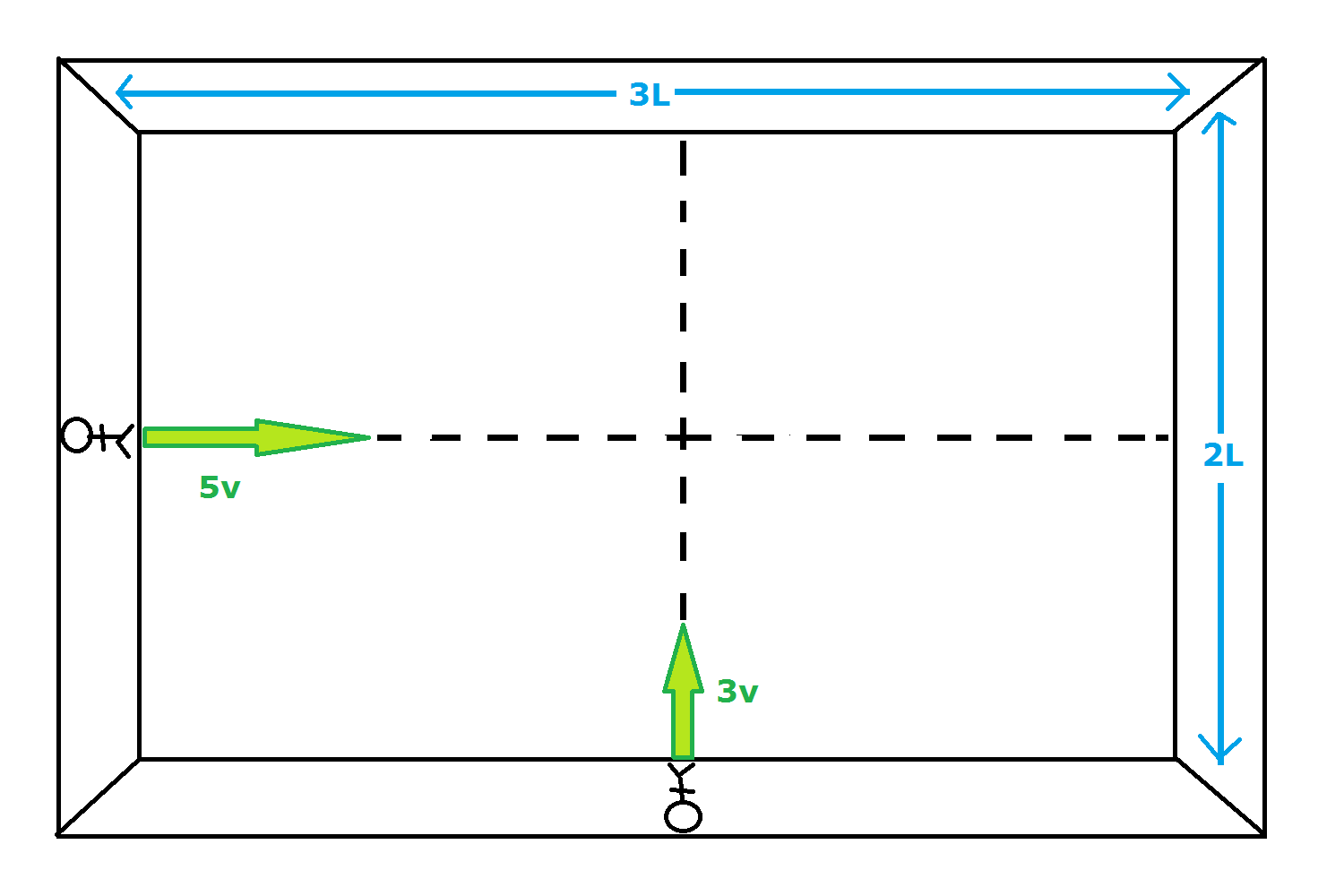# Crossing Paths in a Space StationTwo astronauts, Alfred and Bob, are positioned at the midpoints of adjacent walls, in a rectangular room, in a space station. Alfred's wall has length $3L$, and Bob's wall has length $2L$.

They both push off of their walls at the same instant. Alfred has a speed of $3v$, and Bob has a speed of $5v$. They both stop when they reach their opposing walls.

The closest distance they ever get to each other can be written as $\frac{L}{a\sqrt{b}}$, where $a$ and $b$ are positive integers and $b$ is square free.

Find $a+b$.

Ignore gravity and air resistance.

×